#### You may also like### Days and Dates

Investigate how you can work out what day of the week your birthday will be on next year, and the year after...### Summing Consecutive Numbers

15 = 7 + 8 and 10 = 1 + 2 + 3 + 4. Can you say which numbers can be expressed as the sum of two or more consecutive integers?### Always the Same

Arrange the numbers 1 to 16 into a 4 by 4 array. Choose a number. Cross out the numbers on the same row and column. Repeat this process. Add up you four numbers. Why do they always add up to 34?

# Regular Hexagon Loops

##### Age 11 to 14 Challenge Level:

In all these solutions, $t$ will represent the number of tiles, $I$ the inner perimeter and $O$ the outer perimeter.

Sameer, from Hymers College, was able to observe some patterns in the loops that he observed.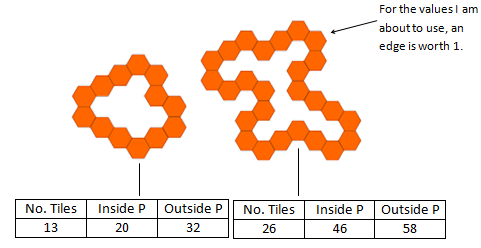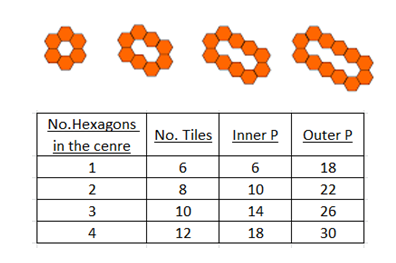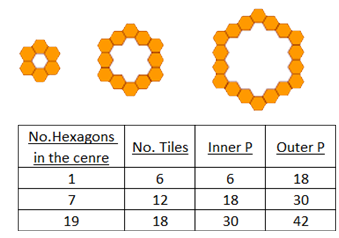If we subtract 3 from the number of tiles and multiply the result by 2, we get the inside perimeter.

If we add 3 to the number of tiles and multiply the result by 2, we get the outside perimeter.

Here are the equations for that:
$\begin{eqnarray} I &=& 2(t-3) \\ O &=& 2(t+3) \end{eqnarray}$

He was also able to make another useful observation:

The difference between the outer perimeter and the inner perimeter is always $12$.

Zach divided the hexagons into three types, depending on where they were in the loop: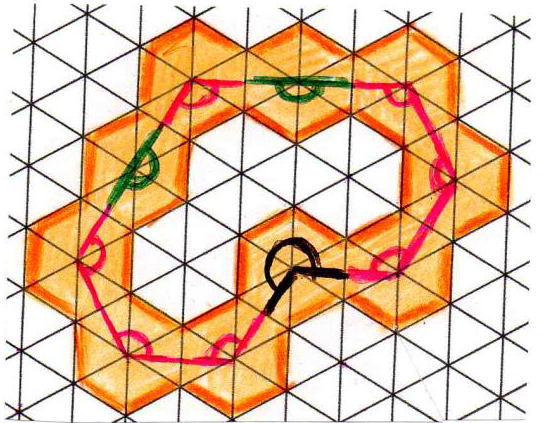$120^\circ$ angles are marked in red, $180^\circ$ angles are marked in green and $240^\circ$ angles are marked in black.

Each $120^\circ$ angle hexagon contributes $1$ side to the inner perimeter, and $3$ sides to the outer perimeter.
Each $180^\circ$ angle hexagon contributes $2$ sides to both the inner and outer perimeters.
Each $240^\circ$ angle hexagon contributes $3$ sides to the inner perimeter and $1$ side to the outer perimeter.

He was then able to use this to count the number of faces in a very long loop: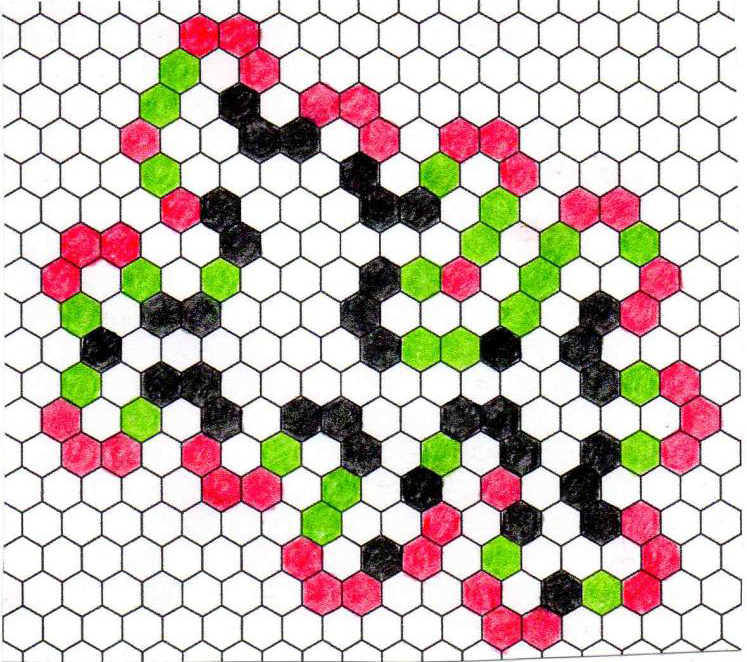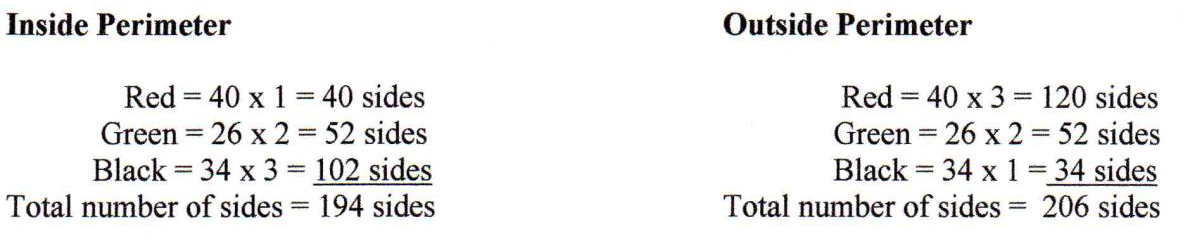Zach was then able to confirm that these agreed with the rules he had found, which were the same as Sameer's

There are 100 hexagons in the loop, so $t = 100$. Then, $2t - 6 = 2 \times 100 - 6 = 194 = I$ and $2t+6 = 2 \times 100 + 6 = 206 = O$.

Raymond, from Greenacres Public School, Australia, provided some formulae from these observations to connect the numbers of the different types of tiles with the inner and outer perimeters.

Let $x$ be the numberof hexagons ONLY on a straight line, not a corner. (These are the $180^\circ$ or green hexagons from Zach's solution).
Let $y$ be the number of corner hexagons curving into the loop. (These are the $240^\circ$ or black hexagons from Zach's solution).
Let $z$ be the number of other corner hexagons. (These are the $120^\circ$ or red hexagons from Zach's solution).

Then:
$O = 2x + y + 3z$

$I = 2x + 3y + z$

Thank you to all of you for submitting your solutions - well done!

Since the hexagons must form a loop, there must be exactly six more outward ($120^\circ$ or red) hexagons than there are inward ($240^\circ$ or black) hexagons. You can check that this is true for both of Zach's examples. This happens because it takes six $120^\circ$ angles to form a loop, and each $240^\circ$ angle cancels one of these out, so there must be exactly six more.

This means $z=y+6$.

Also, as all the hexagons are of these three types, we know that $t = x+y+z$

Therefore:
$O = 2x + y + 3z = 2t + z - y = 2t + 6$
$I = 2x + 3y + z = 2t +y -z = 2t - 6$

These are exactly the patterns that Sameer noticed at the beginning! Now we know that these will always happen.